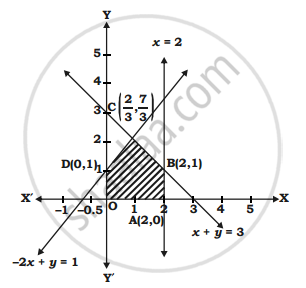Share

# Solve the following LPP by using graphical method. Maximize : Z = 6x + 4y - Mathematics and Statistics

#### Question

Solve the following LPP by using graphical method.

Maximize : Z = 6x + 4y

Subject to x ≤ 2, x + y ≤  3, -2x + y ≤  1, x ≥  0, y ≥ 0.

Also find maximum value of Z.

#### Solution

 Inequalities x ≤ 2 x+y≤3 -2x+y≤1 Equalities x=2 x+y=3 -2x+y=1 Intercept form x/2=1 x/3+y/3=1 x/(-1/2)+y/1=1 Origin Test 0≤2 0+0≤3 -2(0)+0≤1 True Origin Side True Origin Side True Origin SideShaded portion OABC is the feasible region, Where O(0,0) A(2, 0) D(0, 1), B(2, 1)

For C :

x + y = 3

– 2x + y = 1

–   –      –

----------------------

3x = 2

∴ x = 2/3

2/3+y=3 i.e y=7/3

∴ c(2/3,7/3)

Z = 6x + 4y

Z at O(0, 0) = 6(0) + 4(0) = 0

Z at A(2, 0) = 6(2) + 4(0) = 12

Z at B(2, 1) = 6(2) + 4(1) = 16

Z at c(2/3,7/3)=6(2/3)+(7/3)4=40/3

Z at D(0,1) = 6(0) + 4(1) = 4 Thus, Z is maximized at B(2, 1) and its maximum value is 16.

Is there an error in this question or solution?